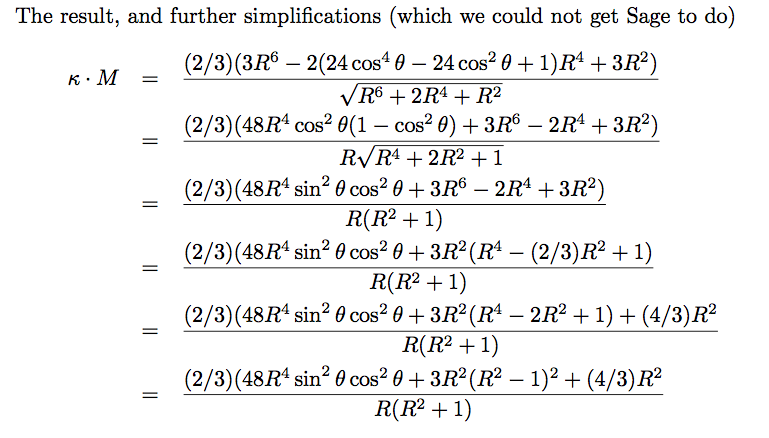# can sage be made to do this algebra?

Enneper's wire lies on an ellipsoid. I want to prove the curvature vector of Enneper's wire always points inward (never tangent to the ellipsoid). The plan is to show that the dot product of the curvature vector with the inward normal to the ellipse is strictly positive.

So I start like this:

  R,t = var('R,t')
X = vector((R * cos(t) - (1/3)* R^3 * cos(3*t),
- R * sin(t) - (1/3) *R^3 * sin(3*t),
R^2 *cos(2*t)))
Xtheta = X.diff(t)
T = 1/(abs(Xtheta)) * Xtheta   # unit tangent
kappavector = T.diff(t)
EllipsoidNormal = vector((-2*R*cos(t) + (2/3)*R^3*cos(3*t),
2*R*sin(t) + (2/3)*R^3 * sin(3*t),-(4/3)*R^2*cos(2*t)))
test = EllipsoidNormal.dot_product(kappavector).trig_simplify()
print(test)


So far so good.In general it is difficult to get Sage to do something to PART of a formula. For example in sin(4t) + sin(2t), get Sage to apply a double angle formula to sin(4t) so everything comes out in trig functions of 2t.

edit retag close merge delete

You should format your code: Edit your message, select the code, and click the "code" button (the one made of 6 bits).

The originally posted code gave a negative result due to an extra minus sign.
The image of the results came from the code without that minus sign, so it's positive. Thanks for pointing out how to include code and images. Putting assumptions in doesn't make any difference (I did have some in but deleted them for simplicity). So the answer is, to simplify subexpressions you can cut and paste them, simplify them separately, then use substitution and a second cut-and-paste to get them substituted back in. Fine, if you are working interactively, but that doesn't work in a script. Can anyone give a script that starts with sin(4t) + sin(2t) and produces a result that is trig functions of 2*t without pre-calculating the answer (either by hand or in Sage)?

Sort by » oldest newest most voted

Here is a procedure that yields to trig functions of 2*t only:

sage: s = sin(4*t) + sin(2*t)
sage: var('u')
u
sage: s.subs(t=u/2)
sin(2*u) + sin(u)
sage: s.subs(t=u/2).expand_trig()
2*cos(u)*sin(u) + sin(u)
sage: s.subs(t=u/2).expand_trig().subs(u=2*t)
2*cos(2*t)*sin(2*t) + sin(2*t)
sage: s.subs(t=u/2).expand_trig().subs(u=2*t).factor()
(2*cos(2*t) + 1)*sin(2*t)

more

To replace an subexpression inside a sum or a product you can do

sage: t = SR.var('t')
sage: formula = sin(2*t) + sin(4*t)
sage: formula.subs({sin(2*t): t**3 + 2})
t^3 + sin(4*t) + 2


And in your expression there are values of R and t so that the result seems to be negative

sage: test.subs(R=1,t=1.)
-4.64062057506056


But it is not so bad as it seems that all substitutions are actually negative

sage: all(test.subs(R=x, t=y) < 0 for x in srange(0.1,2,0.2) for y in srange(0,4,0.2))
True


You can easily see that the sign is actually determined by the sub expression which can further be divided by R^2

sage: sub_expr = 3*R^6 - 2*(24*cos(t)^4 - 24*cos(t)^2 + 1)*R^4 + 3*R^2
sage: sub_expr2 = (sub_expr / R^2).simplify_rational()
sage: print sub_expr2
3*R^4 - 2*(24*cos(t)^4 - 24*cos(t)^2 + 1)*R^2 + 3


To simplify you can replace cos with a new variable u

sage: u = SR.var('u')
sage: sub_expr3 = sub_expr2.subs({cos(t): u})
sage: print sub_expr3
3*R^4 - 2*(24*u^4 - 24*u^2 + 1)*R^2 + 3


But as you already mentioned this is wrong

sage: bool(sub_expr3 >= 0)
False


One thing that you need to do is to specify domain of variables

sage: assume(R, 'real')
sage: assume(u, 'real')
sage: assume(R > 0)
sage: assume(-1 <= u <= 1)
sage: assumptions()
[R > 0, t is real, R is real, u is real, u >= -1, u <= 1]


But still

sage: bool(sub_expr3 >= 0)
False


But now you sub_expr3 are polynomials of degree 2 (in R^2 and u^2) and you should be able to figure out the sign.

more